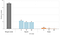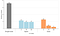# The correctionOriginal (incorrect) Dask runtimesNew (correct) Dask runtimes

# What went wrong

`from sklearn.pipeline import Pipelinefrom sklearn.linear_model import ElasticNetfrom sklearn.compose import ColumnTransformerfrom sklearn.preprocessing import StandardScaler, OneHotEncoderpipeline = Pipeline(steps=[    ('preprocess', ColumnTransformer(transformers=[        ('num', StandardScaler(), numeric_feat),        ('cat', OneHotEncoder(handle_unknown='ignore',                 sparse=False), categorical_feat),    ])),    ('clf', ElasticNet(normalize=False, max_iter=100)),])`
`from dask_ml.compose import ColumnTransformerfrom dask_ml.preprocessing import StandardScaler, DummyEncoder, Categorizerpipeline = Pipeline(steps=[    ('categorize', Categorizer(columns=categorical_feat)),    ('onehot', DummyEncoder(columns=categorical_feat)),    ('scale', ColumnTransformer(        transformers=[('num', StandardScaler(), numeric_feat)],    )),    ('clf', ElasticNet(normalize=False, max_iter=100)),])`
`('scale', ColumnTransformer(        transformers=[('num', StandardScaler(), numeric_feat)],))`
`('scale', ColumnTransformer(        transformers=[('num', StandardScaler(), numeric_feat)],        remainder='passthrough',))`

# All benchmarks are wrong, but some are useful

Experimenter, content creator, data person💡📊💬 rikturr.com

## More from Aaron Richter

Experimenter, content creator, data person💡📊💬 rikturr.com